The average velocity gained by the free electrons of a conductor in the opposite direction of the externally applied electric field is called Drift Velocity. The parameter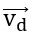is called drift velocity of electrons.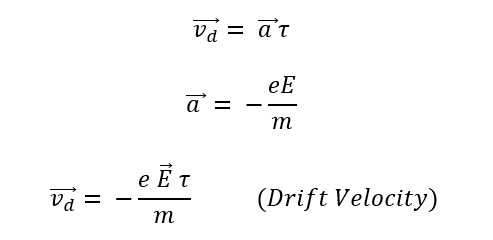## Derivation of OHM’S Law:

Current in terms of drift velocity (vd) is I = enAvd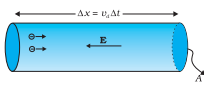Number of electrons in length l of the conductor = n × volume of the conductor = n Al

Total charge contained in length l of the conductor is q = en Al

All the electrons which enter the conductor at the right end will pass through the conductor at the left end in time,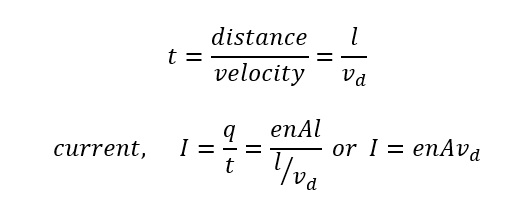This equation relates the current I with the drift velocity vd

Current density ‘j’ is given by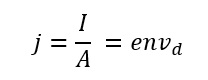In vector form,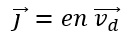The above equation is valid for both positive and negative values of q.

### Deduction of Ohm’s Law:

when a potential difference V is applied across a conductor of length l, the drift velocity in terms of V is given byIf the area of cross section of the conductor is A and the number of electrons per unit volume of the electron density of the conductor is n, then the current through the conductor will beAt a fixed temperature, the quantities m, l, n, e, τ and A, all have constant values for a given conductor.

Therefore,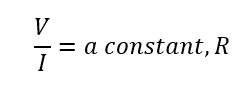This prove Ohm’s law for a conductor and here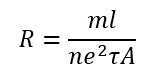is the resistance of the conductor.

### Resistivity in terms of electron density and relaxation time:

The resistance R of a conductor of length l, the area of cross-section A and resistivity ρ is given by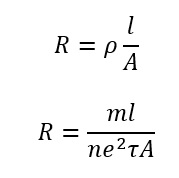where τ is the relaxation time. Comparing the above two equations, we get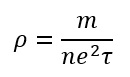Constant value e = 1.6 × 10-19C.

Obviously, ρ is independent of the dimension of the conductor but depends on its two parameters:

1. The number of free electrons per unit volume or electron density of the conductor.
2. The relaxation time τ, the average time between two successive collisions of an electron.

Units:

Drift velocity vd is in ms-1 , free-electron density in m3, cross-sectional area A in m2, current density j in Am-2. All resistance in Ω.